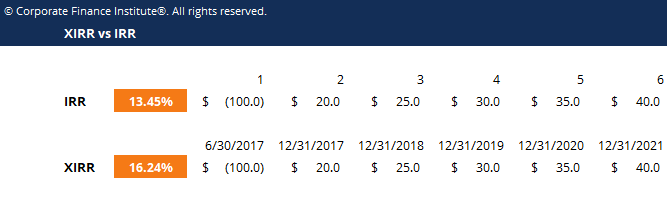# XIRR vs IRR Template

## XIRR vs IRR Template

This XIRR vs IRR template allows you to differentiate between the use of IRR and XIRR functions to compute the internal rate of return.

Below is a screenshot of the XIRR vs IRR template:### XIRR vs IRR Template

The Internal Rate of Return is the discount rate which sets the Net Present Value (NPV) of all future cash flow of an investment to zero.  If the NPV of an investment is zero it doesn’t mean it’s a good or bad investment, it just means you will earn the IRR (discount rate) as your rate of return.

If you use the =IRR() formula in Excel you are using equal time periods between each cell (cash flow).  This makes it challenging when you expect to enter an investment in the middle of a year.

If you use the =XIRR() formula in Excel you have complete flexibility over the time periods of the cash flows.  In order to do this, enter two series in your formula:

• The series of cash flows
• The corresponding dates of each of the cash flows

XIRR gives you the flexibility to assign specific dates to each individual cash flow, making it a much more accurate calculation.

### More Free Templates

For more resources, check out our business templates library to download numerous free Excel modeling, PowerPoint presentation and Word document templates.

• Excel Modeling Templates
• PowerPoint Presentation Templates
• Transaction Document Templates

### Financial Analyst Certification

Become a certified Financial Modeling and Valuation Analyst (FMVA)® by completing CFI’s online financial modeling classes and training program!